# KSEEB Solutions for Class 8 Maths Chapter 15 Quadrilaterals Additional Questions

Students can Download Maths Chapter 15 Quadrilaterals Additional Questions and Answers, Notes Pdf, KSEEB Solutions for Class 8 Maths helps you to revise the complete Karnataka State Board Syllabus and score more marks in your examinations.

## Karnataka State Syllabus Class 8 Maths Chapter 15 Quadrilaterals Additional Questions

Question 1.
Complete the following
a. A quadrilateral has four sides.
b. A quadrilateral has two diagonals
c. A quadrilateral in which one pair of sides are parallel to each other is trapezium.
d. In an isosceles trapezium the base angles are equal.
e. In a rhombus, the diagonals bisect each other in right angles.
f. In a square, all the sides are equal.

Question 2.
Let ABCD be a parallelogram, what special name will you give if.
a. AB = BC
Solution:
Rhombus

Solution:
Rectangle

Solution:
SquareQuestion 3.
A quadrilateral has three acute angles each measuring 70°. What is the measure of the fourth angle?
Solution:
Measure of the fourth angle
= 360 – (70 + 70 + 70) = 360 – 210
= 140°

Question 4.
The difference between two adjacent angles of a parallelogram is 20° . Find the measures of all the angles ‘ of the parallelogram.
Solution:
Let the adjacent angles be ‘x’ and ‘y’
x + y = 180° [Adjacent angles are supplementary]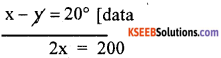x = $$\frac{200}{2}$$ ; x = 100°
x + y = 180
100 + y = 180°
y = 180 – 100
y = 180°
∴The angles are 100°, 80°, 100° and 80°Question 5.
The angles of a quadrilateral are in the ratio 1 : 2 : 3 : 4 find all the angles.
Solution:
Ratio of the angles 1 : 2 : 3 : 4
Let the angles be x, 2x, 3x and 4x
x + 2x + 3x + 4x = 360°
[Angle sum property of a quadrilateral]
10x = 360°
x = $$\frac{360}{10}$$ ; x = 36°
2x = 2 x 36 = 72°, 3x = 3 x 36 = 108°
4x = 4 x 36 = 144°
∴ The angles are 36°, 72°, 108° and 144°

Question 6.
Let PQRS be a parallelogram with PQ = 10 cm and QR = 6 cm. Calculate the measures of the other two sides and measures of the other two sides and perimter of PQRS.
Solution:
PQ = RS = 10 cm
[opposite sides of a parallelogram]
QR = SP = 6 cm
Perimeter = PQ + QR + RS + SP
= 10 + 6 + 10 + 6
= 32 cm

Question 7.
The perimeter of a square is 60 cm. Find the length of its side.
Solution:
Perimeter of a square = 4a
60 = 4a
∴ a = $$\frac{60}{4}$$ a = 15 cm
The length of the side is 15 cmQuestion 8.
Let ABCD be a square and let AC = BD = 10 cm let AC and BD intersect at O. Find OC and OD.
Solution: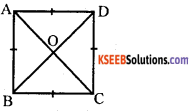The diagonals of a square bisect each other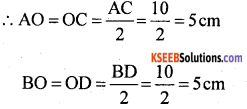Question 9.
Let PQRS be a rhombus with PR = 15 cm and QS = 8 cm. Find the area of the rhombus.
Solution:
Area of rhombus PQRS = Area of ∆ PQS + Area of ∆ RQS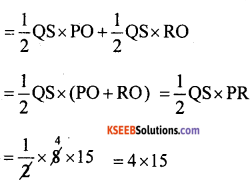Area of rhombus = 60 cm2Question 10.
Let ABCD be a parallelogram and suppose the bisectors of ∠A and ∠B meet at P prove that ∠APB = 90°
Solution: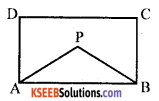∠A + ∠B = 180°
$$\frac{1}{2}$$∠A + $$\frac{1}{2}$$ ∠B = ∠B . 180° [Multiplying by $$\frac{1}{2}$$ ]
∠PAB + ∠PBA = 90° [AP and BP are angular bisectors]
∠PAB + ∠PBA +∠APB = 180° [Angle sum property of a triangle]
90 +∠APB = 180°
∠APB = 180 – 90
∠APB = 90°Question 11.
Let ABCD be a sqaure. Locate points P, Q, R, S on the sides AB, BC, CD, DA respectively such that AP = BQ = CR = DS. Prove that PQRS is a square.
Solution:
Let AP = BQ = CR = DS = xAP + PB = AB
x + PB = AB
PB = AB – x
AB = BC
∴ QC = AB – x
In ∆ PBQ and ∆ QCR
BQ = CR[= x]
BP = QC [AB – x]
∠B = ∠C [= 90°]
∴ ∆ PBQ = A QCR [SAS postulate]
∴ PQ = QR
[corresponding sides] similarly we can show that QR = SR and SR = SP
i.e., PQ = QR = RS = SP …… (i)
∠BQP = ∠CRQ [corresponding angles]
∠BQP + ∠PQR + ∠RQC = 180° [Angle sum property of a triangle]
∠BQP + ∠PQR + 90 – ∠CRQ = 180°
[∴ ∠CQR = 90 – ∠CRQ]
∠BQP + ∠PQR – ∠BQP = 180 – 90
[∵ ∠DQP = ∠CRQ]
∠PQR = 90°
∴ PQRS is a square.Question 12.
Let ABCD be a rectangle and let P, Q, R, S be the midpoints of AB, BC, CD, DA respectively prove that PQRS is a Rhombus.
Solution:
In ∆ APS and ∆ CQR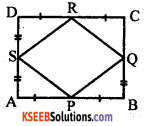AP = CR [P and R are the midpoints of AB and CD)
AS = CQ [S and Q are the midpoints of AD and BC]
∠SAP = ∠RCQ [=90° angles of a rectangle]
∴ ∆ APS ≅ ∆ CQR [SAS postulate]
∴ SP = QR [corresponding sides] similarly we can show PQ = QR = RS
i.e., PQ = QR = RS = SP
∴ PQRS is a rhombus

Question 13.
Let ABCD be a quadrilateral in which diagonals intersect at ‘O’ perpendicularly. Prove that AB + BC + CD + DA > (AC + BD)
Solution:
In A AOD, AD > AO + OD … (i)
In A AOB, AB > AO + OB … (ii)
In A BOC, BC > BO + OC … (iii)
In A COD, CD > DO + OC … (iv)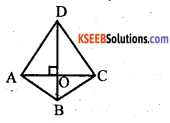By adding (i), (ii), (iii) and (iv)
AD + AB + BC + CD > AO + OD + AO + OB + BO + OC + DO + CO > 2 AO + 2 OC + 2 OB + 2 OD > 2 (AO + OC + OB + OD)
AD + AB + BC + CD > 2 (AC + BD) [∵ AO + OC = AC OB + OD = BD]
∴ AD + AB + BC + CD > AC + BDQuestion 14.
Let ABCD be a quadrilateral with diagonals AC and BD. Prove the follwoing statements (compare these with the previous problem)
a. AB + BC + CD > AD
b. AB + BC + CD + DA > 2AC
c. AB + BC + CD + DA > 2BD
d. AB + BC + CD + DA > AC + BD
Solution:
a. AB + BC + CD + DA > AC + BD [From previous problem]
AB + BC + CD > AC + BD – AD
∴ AB + BC + CD > – AD

b. AB + BC + CD + DA > 2 (AC + BD) [From the previous problem]
AB + BC + CD + DA > 2AC + 2BD
∴ AB + BC + CD + DA > 2AC

c. AB + BC + CD + DA > 2AC + 2BD [From the previous problem]
∴ AB + BC + CD + DA > 2BD

d. AB + BC + CD + DA > AC + BD [From the previous problem]

Question 15.
Let PQRS be a kite such that PQ > PS. Prove that ∠PSR > ∠PQR (Hint : Join QS)
Solution: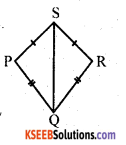Join QS
In ∆ PQS, PQ > PS [data]
∴ ∠PSQ > ∠PQS …(i)
In ∆ SRQ, RQ > SR
[∵ PQ = QR and SP = SR]
∴ ∠QSR. > ∠SQR … (ii)
∠PSQ + ∠QSR > ∠PQS + ∠SQR
∠PSR >∠PQRQuestion 16.
Let ABCD be a quadrilateral in which AB is the smallest side and CD is a
largest side ∠A > ∠C and ∠B > ∠D ( Hint: join AC and BD)
Solution:Join AC and BD In ∆ ABD, AB is the smallest side
∠ABD > ∠APB
[Angle opposite to larger side] …(i)
In ∆ CBD, CD is the largest side
∴ CD > CB
∴ [CBD > ∠CDB [Angle opposite to larger side] …. (ii)
By adding (i) and (ii) .
∠ABD + ∠CBD >∠ADB + ∠CDB
∴ ∠B >∠D
In ∆ ABC, AB is the smallest side] ……. (iv)
∴ BC > AB ∠BAC > ∠ACB [Angle opposite to larger side] … (iii)
In ∆ ADC, CD is the largest side
∠CAD > ∠ACD [Angle opposite to larger side] …(iv)
∠BAC + ∠CAD > ∠ACB + ∠ACD
∠A > ∠CQuestion 17.
In a triangle ABC, let D be the midpoint of BC. Prove that AB + AC > 2 AD (what property of quadrilateral is needed here)
Solution:
In ∆ ABD, AB + BD > AD … (i)
In ∆ ACD, AC + DC > AD …(ii)
AB + AC + BD + DC > 2AD
AB + AC + BC > 2AD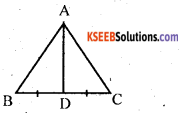orQuestion 18.
Let ABCD be a quadrilateral and let P, Q, R, S be the midpoints of AB, BC, CD, DA respectively prove that PQRS is a parallelogram [what extra result you need to prove this] It can be proved if ABCD is a rectangle
Solution:
In ∆ APS and ∆ CQR.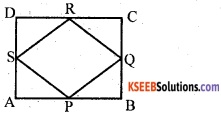AP = CR [P and R are the midpoints of AB and CD]
AS = CQ [∵ S and Q are the midpoints of AD and BC]
∠SAP = ∠RCQ [= 90° angles of a rectangle]
∴ ∆ APS ≅ ∆ CQR [SAS posltulate]
∴ SP = QR [corresponding sides]
similarly we can show PQ = QR = RS
i.e., PQ = QR = RS = SP
∴ PQRS is a rhombus
∴ PQRS is a parallelogram.Question 19.
Prove that the base angles of an isosceles trapezium are equal.
Solution:
In trap ABCD, Draw ⊥rs to base
$$\overline{\mathrm{BE}}$$ and $$\overline{\mathrm{CF}}$$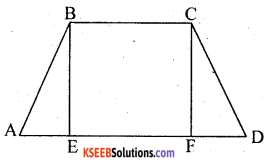$$\overline{\mathrm{BE}}$$ ≅ $$\overline{\mathrm{CF}}$$ (∵ Altitudes of trapezium)
$$\overline{\mathrm{AB}}$$ = $$\overline{\mathrm{CD}}$$ (Given)
$$\overline{\mathrm{AE}}$$ = $$\overline{\mathrm{FD}}$$ (Sides of Right angle)
From SSS congruence
∠A ≅ ∠D [CPCT]

Question 20.
Suppose in a quadrilateral ABCD, AC = BD and AD = BC. Prove that ABCD is a trapezium.
Solution:
Data: ABCD is a quadrilateral in which
AD = BC and AC = BD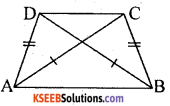To Prove: ABCD is a trapezium
Proof: In ∆ ADB and ∆ ACB AB = AB (Common side)
BD = AC (data)
∴ ∆ ADB ≅ ∆ ACB (SSS postulate)
∴ ∠DAB = ∠ABC (CPCT) …(i)
In ∆ ADC and ∆ BDC
DC = DC (common side)
AC = BD (data)
∴ ∆ ADC ≅ ∆ BDC (SSS postulate)
∴ ∠ADC = ∠BCD (IPCT) …(ii)
∠ABC + ∠ABC + ∠BCD + ∠BCD = 360°
[∠ABC = ∠DAB, ∠ADC = ∠BDC] 2∠ABC + 2∠DCD = 360°
2 (∠ABC + ∠BCD = 360°)
∠ABC +∠BCD = $$\frac{360^{\circ}}{2}$$ = 180°
But theSe are co-interior angles AB || DC
∴ ABCD is a trapezium.Question 21.
Prove logically the diagonals of a parallelogram bisect each other. Show conversely that a quadrilateral in which diagonals bisect each other is a parallelogram.
Solution:
Given: A parallelogram ABCD with diagonal AC and BD intersecting at O.
To prove: AO = OC and BO = OD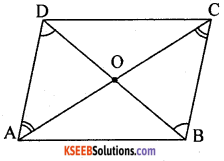Proof: Consider ∆ AOD and ∆ COB.
We observe
∠DAO = ∠BCO (alternate angles)
AD = BC (opposite sides of a parallelogram)
By ASA, ∆ AOD ≅ ∆ COB.
Hence AO = OC and OD = OB
Given: a quadrilateral ABCd such that AC and BD bisect each other.
To prove: ABCD is a parallelogram.
Proof: Let AC and BD intersect at K.
In triangles AKD and CKB, we observe
AK = KC (given)
BK = KD (given)
∠AKD = CKB (vertically opposite angles)Hence by SAS congruency,
∆ AKD ≅ ∆ CKB. lt follows that
∠DAK = ∠BCK and AD = CB.
Thus AD || BC. Similarly, we can prove AB || CD and AB = CD.
Therefore ABCD is a parallelogram.Question 22.
Prove logically that the diagonal of a rectangle are equal.
Solution:
In rectangle ABCD, AC and BD are diagonal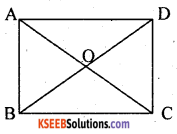In ∆ AOD and ∆ BOC
∠AOD = ∠BOC (V.O.A)
∠DAO = ∠BCQ (Alternate angle]
∴ ∆ AOD ≅ ∆ BOC OA = OC, OB = OD
In ∆ AOB and ∆ COD
∠AOB = ∠COD (V.O.A)
∠OAB = ∠PCD (Alternate Angle)
∠QBA = ∠ODC (Alternate Angle)
∆ AOB ≅ ∆ COD
∴ OA = OC, OB = OD
∴ Diagonal AC = Diagonal BD.Question 23.
In a rhombus PQRS, ZSQR = 40° and PQ = 3 cm. Find ZSPQ, ZQSR and the perimeter of the rhombus.
Solution:
Given PQRS is a rhombus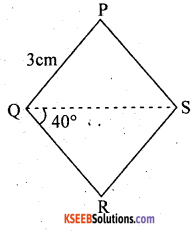∠SQK = 40°, PQ = 3cm
Perimeter of rhombus PQRS
= PQ + QR + SR + SP 3 + 3 + 3 + 3 = 12 cm

Question 24.
Let ABCD be a rhombus and ∠ABC = 124°. Calculate. ∠A, ∠D and ∠C.
Solution: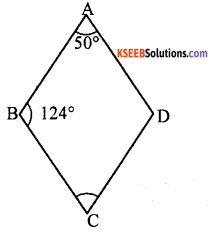In a rhombus ABCD,
If ∠ABC = 124° then
∠ADC = 124° (Opposite angles are equal)
∴ ∠A = 56°, ∠D = 124°, ∠C = 56°Question 25.
Four congruent rectangles are placed as shown in the figure. Area of the outer square is 4 times that of the inner square is 4 times that of the inner square. What is the ratio of length to breadth of the congruent rectangles?
Solution: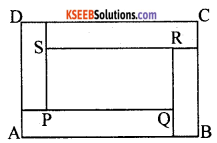Outer square
ABCD = 4 × Inner square PQRS

Question 26.
Let ABCD be a quadrilateral in which ∠A = ∠C, and ∠B = ∠D. Prove that ABCD is a parallelogram.
Solution:
ABCD is a Quadrilateral in which AC and BD are the diagonals intersecting Each other at O.
OA = OC, and OB = OD.
∵ O is the midpoint of diagonals
∴ Each pair of opposite angles are equal. It is a parallelogram.Question 27.
In a quadrilateral ABCD, suppose AB = CD and AD = BC. Prove that ABCD is a parallelogram.
Solution: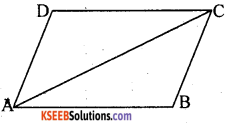Given: Quadrilateral ABCD in which AB = DC and AD = BC
To Prove: ABCD is a parallelogram.
Construction: Draw diagonal AC.
Proof: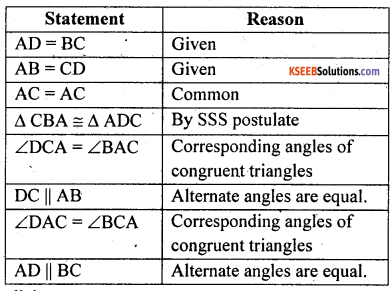∴ ABCD is a parallelogram.